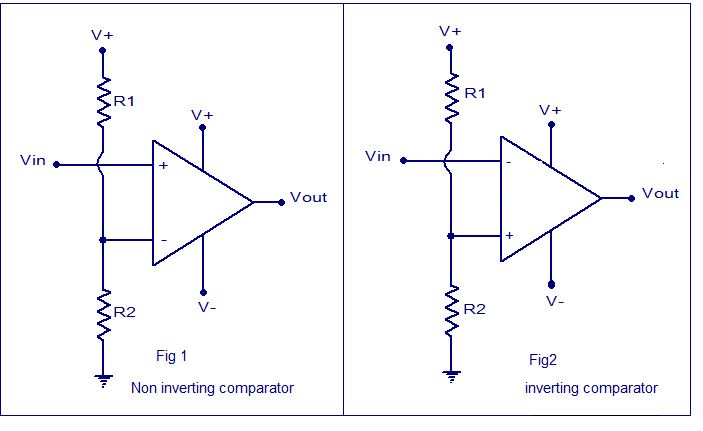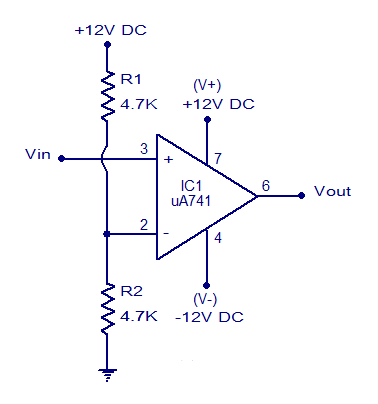## Audio

[Audio][slideshow]

## Voltage comparator circuit.

Voltage comparator is a circuit which compares two voltages and switches the output to either high or low state depending upon which voltage is higher. A voltage comparator based on opamp is shown here. Fig1 shows a voltage comparator in inverting mode and Fig shows a voltage comparator in non inverting mode.Voltage comparator

### Non inverting comparator.

In non inverting comparator the reference voltage is applied to the inverting input and the voltage to be compared is applied to the non inverting input. Whenever the voltage to be compared (Vin) goes above the reference voltage , the output of the opamp swings to positive saturation (V+) and vice versa. Actually what happens is that, the difference between Vin and Vref, (Vin – Vref) will be a positive value and is amplified to infinity by the opamp. Since there is no feedback resistor Rf, the opamp is in open loop mode and so the voltage gain (Av) will be close to infinity. So the output voltage swings to the maximum possible value ie; V+. Remember the equation Av = 1 + (Rf/R1). When the Vin goes below Vref, the reverse occurs.

### Inverting comparator.

In the case of an inverting comparator, the reference voltage is applied to the non inverting input and voltage to be compared is applied to the inverting input. Whenever the input voltage (Vin) goes above the Vref, the output of the opamp swings to negative saturation. Here the difference between two voltages (Vin-Vref) is inverted and amplified to infinity by the opamp. Remember the equation Av = -Rf/R1. The equation for voltage gain in the inverting mode is Av = -Rf/R1.Since there is no feedback resistor, the gain will be close to infinity and the output voltage will be as negative as possible ie; V-.

### Practical voltage comparator circuit.

A practical non inverting comparator based on uA741 opamp is shown below. Here the reference voltage is set using the voltage divider network comprising of R1 and R2. The equation is Vref = (V+/ (R1 + R2)) x R2. Substituting the values given in the circuit diagram into this equation gives Vref = 6V. Whenever Vin goes above 6V, the output swings to ~+12V DC and vice versa. The circuit is powered from a +/- 12V DC dual supply.Voltage comparator using 741

Few other opamp related circuits that you may be interested in.

1. Summing amplifier : The summing amplifer can be used to find the algebraic sum of a given number of signals.

2. Integrator using opamp : For an integrating circuit, the output signal will be the integral of the input signal. For example, a sine wave on integration gives cosine wave, square wave on integration gives triangle wave etc.

3.Inverting amplifier : In an inverting amplifier, the output signal will be an inverted version of the input signal and is amplified by a certain factor.

4.Instrumentation amplifier : This is a type of differential amplifier with additional buffer stages at the input. This results in high input impedance and easy matching. The instrumentation amplifier has better stability, high CMRR, low offset voltage and high gain.

[Lights][grids]